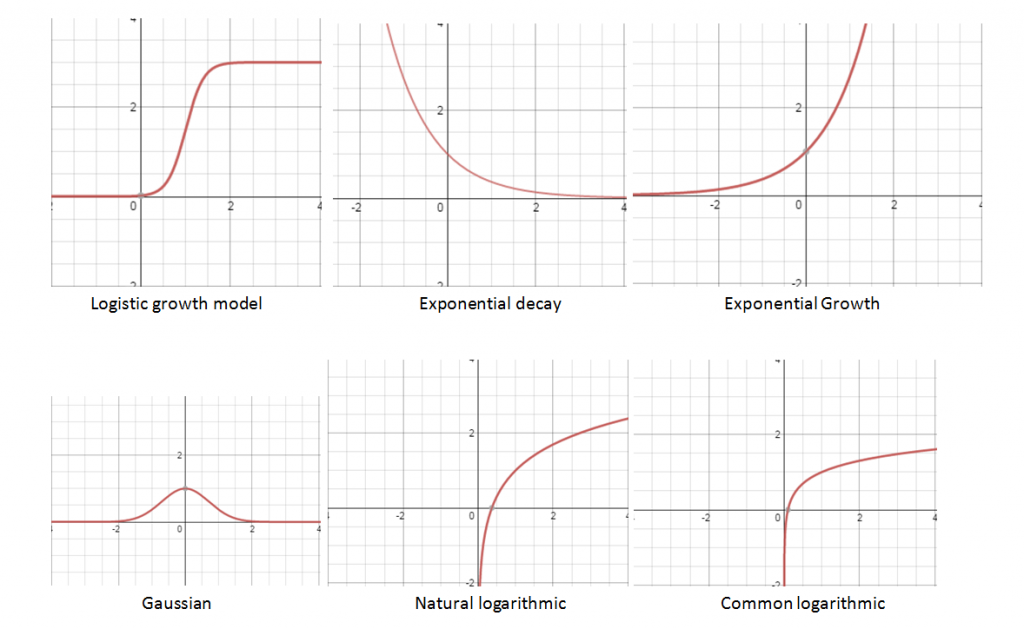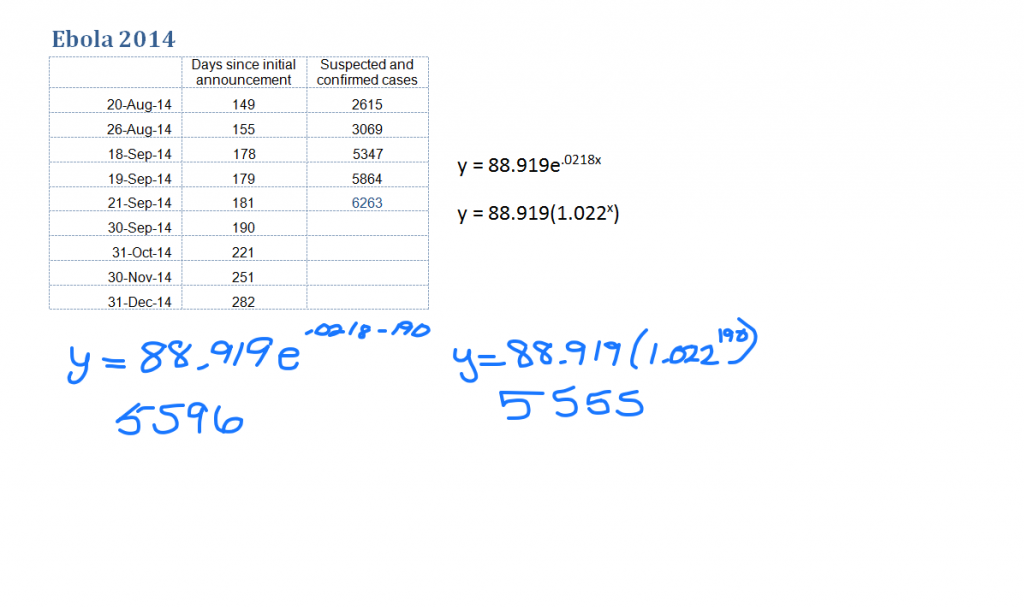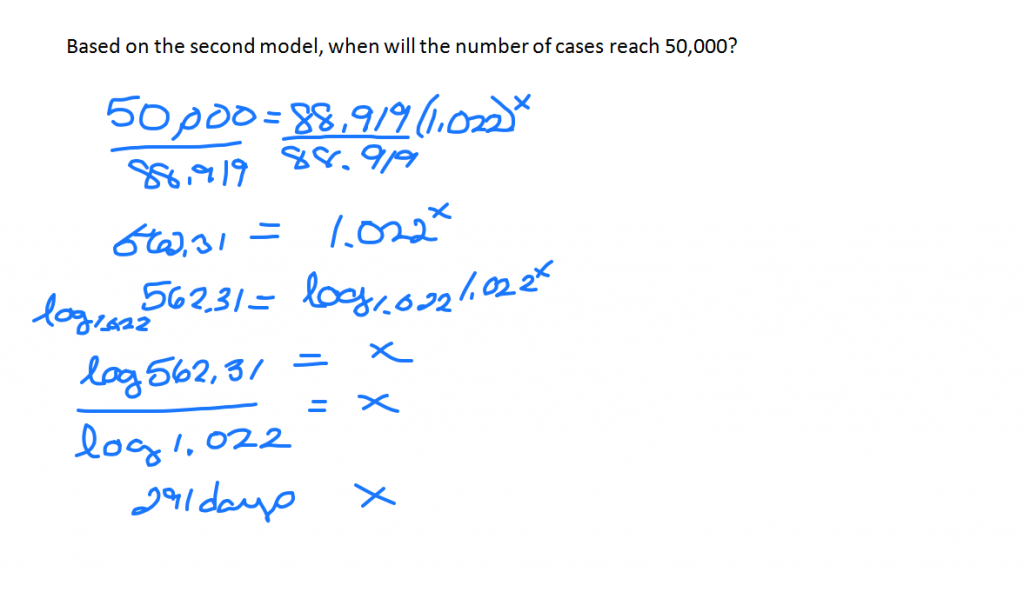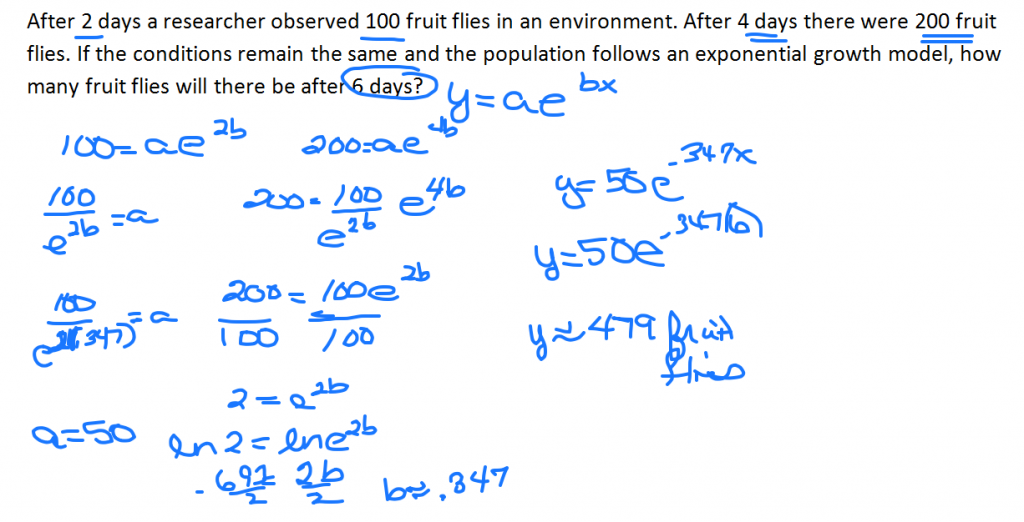# Exponential & Logarithmic Models

//Exponential & Logarithmic Models
Exponential & Logarithmic Models 2017-11-13T22:01:53+00:00There are several models related to exponential and logarithmic functions. A logistics growth model starts out like exponential growth and then tapers off. It is commonly used to model the spread of disease and was used by Sam Walton of Walmart to determine when to take items off the shelf to make room for the next hot selling merchandise. Exponential decay is used in carbon dating and depreciation. Exponential growth is used to model the growth of money and population growth. Gaussian models correspond with bell curves and are often used in academia. The natural and common logarithmic models are used when calculating intensity of earthquakes, sound, and acidity.

At the time of this writing, the current Ebola outbreak data is matching an exponential growth model. What health care workers and statisticians are hoping for is that the data will start to trend toward a logistics model.The CDC estimates that by September 30, 2014 there will be approximately 8,000 cases or as many as 21,000 cases if corrections for under-reporting are made. It estimates that for every Ebola case there are 1.5 times as many unreported cases.